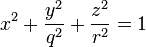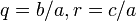# Difference between revisions of "Ellipsoid in three-dimensional Euclidean space"

This surface type is not unique up to isometry or even up to similarity transformations, but rather, depends upon multiple nonzero parameters$a,b,c$. If we're considering the surface up to rigid isometries, the parameters are unique up to permutations. If we're considering the surface up to similarity transformations, the parameters are unique up to the action of permutations and projective equivalence.
Up to rotations$\frac{(x - x_0)^2}{a^2} + \frac{(y - y_0)^2}{b^2} + \frac{(z - z_0)^2}{c^2} = 1$ This version need not be centered along the origin but is oriented parallel to the axes.
Up to rigid motions (rotations, translations, reflections)$\frac{x^2}{a^2} + \frac{y^2}{b^2} + \frac{z^2}{c^2} = 1$ This version is centered at the origin and oriented parallel to the axes.
Up to similarity transformations$x^2 + \frac{y^2}{q^2} + \frac{z^2}{r^2} = 1$ Here,$q = b/a, r = c/a$ For this version, we can normalize one of the three values$a,b,c$. In the form given here, we chose to normalize$a$.